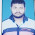## Mathamatice (Subsidiary/General)

Question - 01

- State and Prove Leibnitzs theorem........Answer

If  y1/m+y1/m =2x , Prove that (x2-1) yn+2 +(2n +1) xyn+1 - (n2- m2)yn = 0......Answer (YouTube Video)

- Discuss various methods for solving first order and first degree differential equations.....Answer

- Solve, the equation,  dy/dx + ytanx = y3secx.....Answer

- Explain Principle of Virtual work......Answer

- State the convergence of the series ∑ 4.7 ...(3n+1)/1.2 ...n . Find .....Answer

- Find  the common area to the circles x2 + y2 = 4 and x2 + y2 = 4x......Answer

- State and Prove Keplar's law of planetary motion.....Answer

- Define differentiation of vector point function and show that if r = acoswt +dr bsinwt then r x dr/dt =wa x b and drt/dt =-w°r, where a, b and w are contant......Answer

Note- आपको किस विषय का Assignment चाहिए हमें Comment में बताएं, हम जल्दी उपलब्ध करा देंगे |

1.Bhai buhut buhut thanks bhai help krne ke liye😗

2.Cannot Explain In Words Thanks🙏🏻❤

3.bhai milte naikhe

1.Niman se khoja sab mili

4.5.1.6.7.